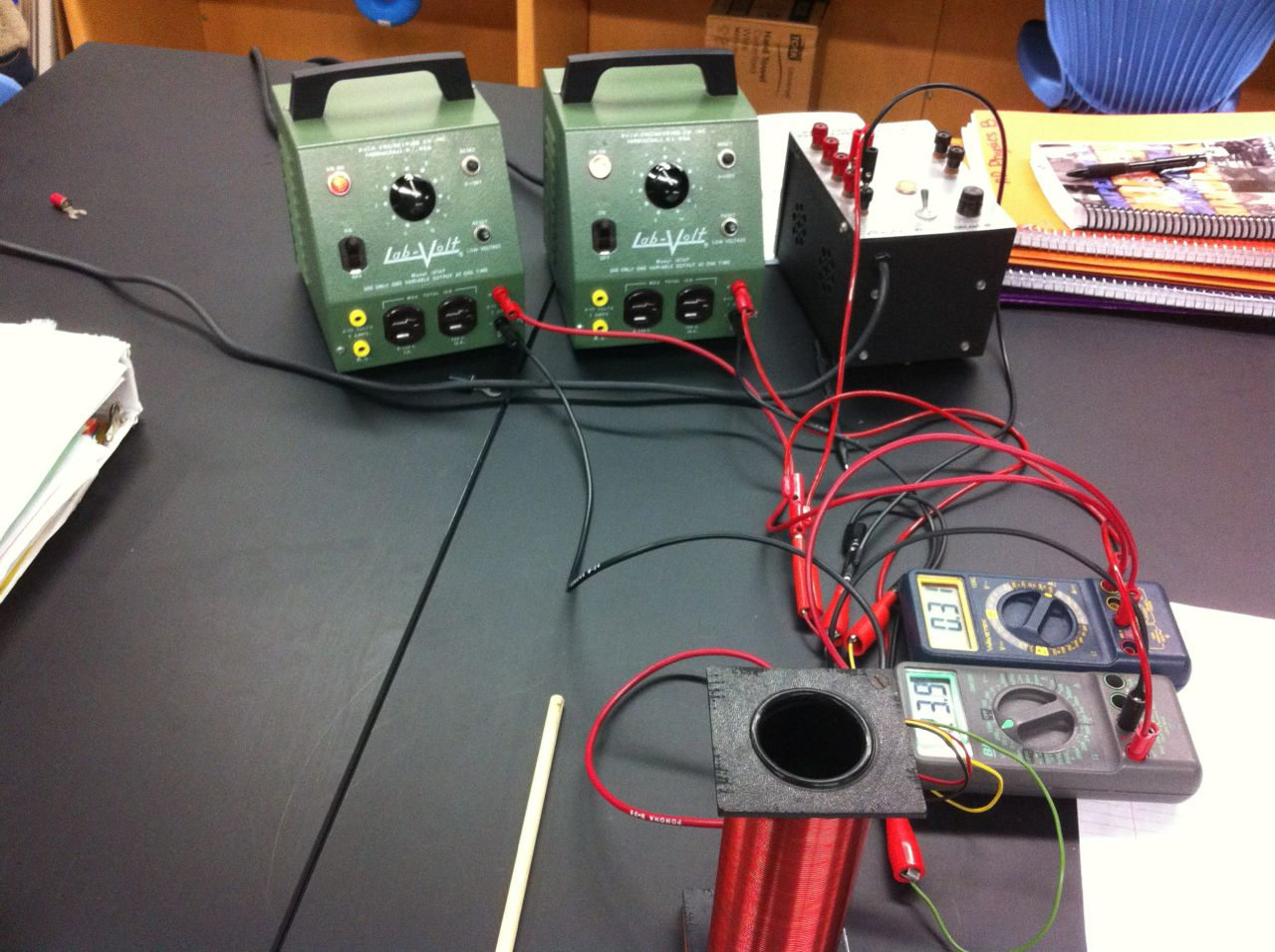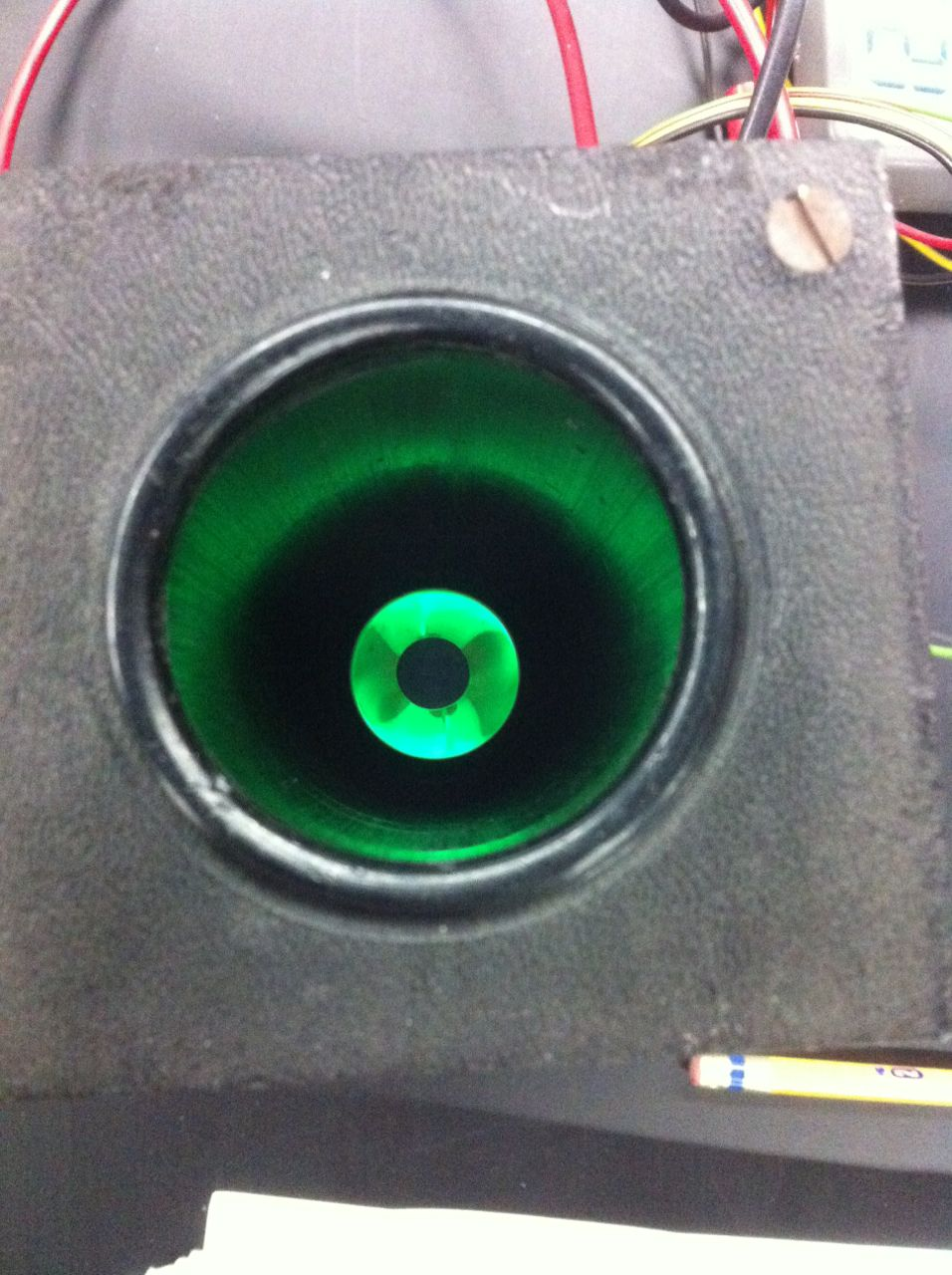# Day 103: Mass of the Electron Lab

Today we did one of my favorite labs in AP Physics B. Students measured the mass of an electron. They placed a vacuum tube in a solenoid to deflect the electron path. They measured the current through the solenoid, the accelerating voltage of the vacuum tube, and the radius of curvature of the electron path. They derived the necessary equations to relate these three variables to the mass of the electron. They use different sized dowels and corks to measure the radius of curvature which isn’t very precise, but we usually are within one or two orders of magnitude of the actual mass.

Here is the experimental setup:Here is the electron beam:##practicumlab ##magnetism